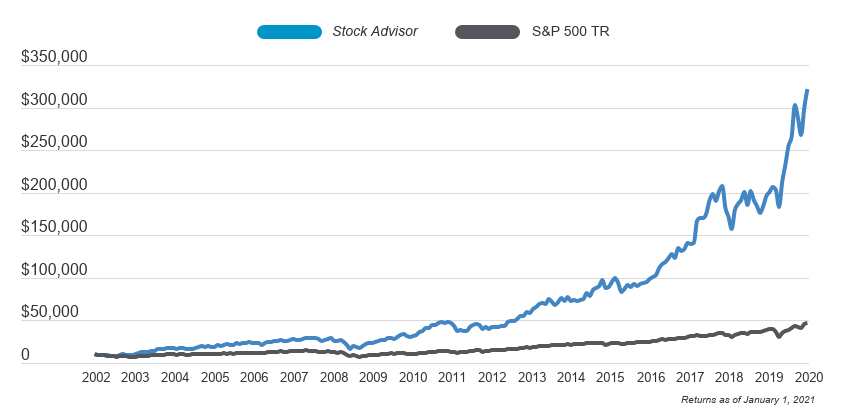## This important bond metric tells you how sensitive a bond is to interest rate changes.In many ways, bonds are more complex than stocks. Investors use a host of different metrics to evaluate bond investments, and one involves a concept known as duration, which helps an investor understand how sensitive a bonds value is to interest rate changes. There are several different types of duration calculations, but one involves whats known as modified duration and produces the percentage change in price for a given change in interest rates.

A two-step process to calculate modified duration
The easiest way to come up with the modified duration for a bond is to start by calculating another type of duration called Macauley duration. This type of duration produces the weighted average time in which the investor will receive cash flows from the bond.

To calculate Macauley duration, you have to figure out the timing of all cash flows from the bond. Most bonds make relatively small interest payments and then make a big principal repayment at maturity.

Once you know how much and when all payments will be made, you have to time-weight their discounted values. To do so, take the present value of each bond payment, discounted by the current yield to maturity. Keeping each present value separate, multiply the present value by the period in which the payment is made. For instance, with a two-year bond paying annual interest payments, youll multiply the present value of the first payment by 1 and the second payment by 2. Then add those numbers together and divide by the present value of all the bonds payments.

For example, say you have a two-year bond paying annual interest at 5% and with a yield to maturity of 5%. Its current price is \$1,000. The present value of the first payment would be (1000 x 5%) / (1 + 5%), or \$47.62. The present value of the second payment, including the maturity amount, would be ((1000 + (1000 * 5%) / (1 + 5%)^2), or \$952.38. So the Macauley duration would be ((\$47.62 x 1) + (\$952.38 x 2)) / (\$47.62 + \$952.38), which works out to 1.952.

To calculate modified duration, you take the answer above and divide it by the sum of 1 and the bonds yield to maturity. So 1.952 / (1 + 5%) = 1.859.

What modified duration means
The modified duration tells you how much the price of a bond will change for a given change in its yield. So in the example above, investors can expect to see a 1.859% move in price when the bonds yield to maturity changes by one percentage point.

In general, the longer the maturity of a bond, the higher its modified duration. Higher-interest bonds tend to have smaller modified durations because more of their cash flow comes from interest payments that come sooner in the bonds lifespan.

Bonds that have high modified durations are especially subject to interest rate risk. When rates are looking to head higher, looking at modified duration is important to understand exactly what could happen in a rising-rate environment.

This article is part of The Motley Fools Knowledge Center, which was created based on the collected wisdom of a fantastic community of investors. Wed love to hear your questions, thoughts, and opinions on the Knowledge Center in general or this page in particular. Your input will help us help the world invest, better! Email us at knowledgecenter@fool.com. Thanks -- and Fool on!

## Motley Fool Returns

619% 141%

Discounted offers are only available to new members. Stock Advisor will renew at the then current list price. Stock Advisor list price is \$199 per year.

Stock Advisor launched in February of 2002. Returns as of 09/01/2021.

Cumulative Growth of a \$10,000 Investment in Stock Advisor Calculated by Time-Weighted Return